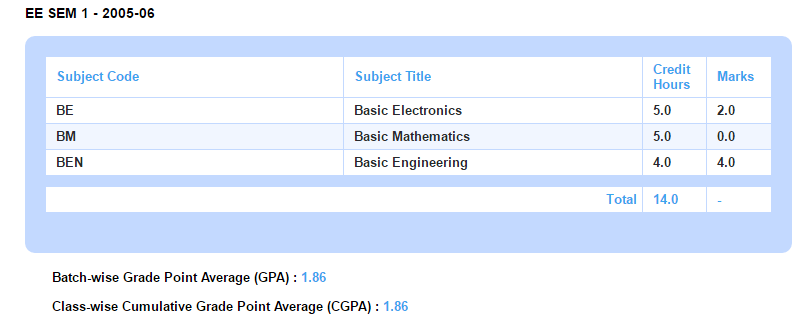# What is Grade Point Average?

Modified on Mon, 27 Jun 2016 at 12:39 PM

Grade Point Average (GPA) is the average of the grade point values obtained by a student for the courses taken in a semester. The average is calculated by assigning a number to the grade a student obtains for each course, and using that number to calculate the student’s GPA.

Course-wise Cumulative Grade Point Average (CGPA) is the average of the grade point values obtained by a student in all batches of a course. In Fedena, CGPA can also be calculated based on Credit Hours.